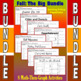# Fall - The Big Bundle - 8 Math-Then-Graph Activities - Solve 2-Step EqsSubject
Resource Type
Product Rating
4.0
1 Rating
File Type

Compressed Zip File

6 MB|40 pages
Share
8 Products in this Bundle
8 products
Bundle Description

Celebrate all your favorite Fall festivities and give students the practice they need at solving 2-step equations and coordinate graphing with these Math-Then-Graph Activities.

In each activity, students are given a list of coordinate points to plot. Some of the points are incomplete. The students need to solve for either x or y in a 2-step equation to complete the point. The students should connect the completed coordinate points only within the designated zones. When they are done, they will have graphed wonderful pictures of Fall.

Here are some tips:

 All points will be on one grid line or another.

 A completed picture has been provided to be used as a key.

 I have also found that printing graph paper directly from themathworksheetsite.com

helps out, but any graph paper will do.

 Remember to copy double-sided when necessary to save paper

Each of these are included but can be purchased separately:

S'more for Me - A Math-Then-Graph Activity - Solve 2-Step Equations

Cider and Donuts - A Math-Then-Graph Activity - Solve 2-Step Equations

Corn Stalk - A Math-Then-Graph Activity - Solve 2-Step Equations

Touchdown - A Math-Then-Graph Activity - Solving 2-Step Equations

Fall Migration - A Math-Then-Graph Activity - Solve 2-Step Equations

Falling Fall Leaves - A Math-Then-Graph Activity - Solve 2-Step Equations

Caramel Apple - A Math-Then-Graph Activity - Solve 2-Step Equations

Fall in the Patch - A Math-Then-Graph Activity - Solve 2-Step Equations

Or purchase multiple activities in one of these other Fall Bundles:

Fall - The Big Bundle - 8 Coordinate Graphing Activities

Fall - The Big Bundle of 8 Quadrant I Coordinate Graphing Activities

Fall - The Big Bundle - 8 Math-Then-Graph Activities - Solve 15 Systems

Fall - The Big Bundle - 8 Math-Then-Graph Activities - Solve 30 Systems

Fall - The Big Bundle - 8 Math-Then-Graph Activities - Finding Vertices

Fall - The Big Bundle - 8 Math-Then-Graph Activities - Solve Matrix Equations

Fall / H'ween / Thxgiving - Crossover-Bundle - 31 Coordinate Graphing Activities

For a Listing of all the Fall Activities:

Same Graph - Different Math: Fall

Also, don't forget to visit my web site:

Mike's Math Market

to see my other Coordinate Graphing Activities

and Math-Then-Graph Activities at affordable prices.

Have Fun!

Total Pages
40 pages